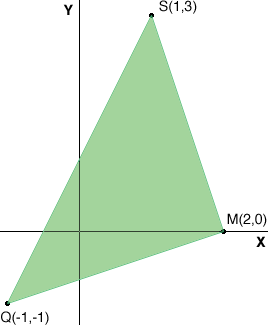SEARCH HOMEMath Central Quandaries & QueriesQuestion from Jessie, a student: find the measures of the sides of triangle KPL and classify each triangle by its sides. my first problem would be K(-3,2) P(2,1) L(-2,-3) ...The three points they give you are the vertices of the triangle and you need to match them up. Draw the triangle and write in the vertices and the related point with the vertex. You will then do the distance formula three times to find the distance of all three sides. Once you have the three sides you can determine if it is scalene, isosceles, or equilateral...using the distance formula how do i solve this?Jessie,

Let me illustrate with different coordinates. Suppose the points are M(2,0), S(1,3) and Q(-1,-1).

1. Draw the triangle and write in the vertices and the related point with the vertex.2. Use the distance formula three times to find the distance of all three sides.

Distance from M to S √[(1 - 2)2 + (3 - 0)2] = √10

Distance from S to Q √[(-1 - 1)2 + (-1 - 3)2] = √20 = 2√5

Distance from Q to M √[(2 -(-1))2 + (0 - (-1))2] = √10

3. Determine if it is scalene, isosceles, or equilateral

Since the lengths of two sides are the same the triangle is isosceles.

I hope this helps,
PennyMath Central is supported by the University of Regina and The Pacific Institute for the Mathematical Sciences.